GeeksforGeeks App
Open AppBrowser
Continue

# Python | Pandas dataframe.rmul()

Python is a great language for doing data analysis, primarily because of the fantastic ecosystem of data-centric python packages. Pandas is one of those packages and makes importing and analyzing data much easier.

Pandas dataframe.rmul() function is used for finding the multiplication of dataframe and other, element-wise (binary operator rfloordiv). This function is essentially same as doing other * dataframe but with a support to substitute for missing data in one of the inputs.

Syntax:DataFrame.rmul(other, axis=’columns’, level=None, fill_value=None)
Parameters :
other : Series, DataFrame, or constant
axis : For Series input, axis to match Series index on
level : Broadcast across a level, matching Index values on the passed MultiIndex level
fill_value : Fill existing missing (NaN) values, and any new element needed for successful DataFrame alignment, with this value before computation. If data in both corresponding DataFrame locations is missing the result will be missing
Returns : result : DataFrame

Example #1: Use rmul() function to find the multiplication of a series with a dataframe.

## Python3

 `# importing pandas as pd``import` `pandas as pd` `# Creating the dataframe``df ``=` `pd.DataFrame({``"A"``:[``1``, ``5``, ``3``, ``4``, ``2``],``                   ``"B"``:[``3``, ``2``, ``4``, ``3``, ``4``],``                   ``"C"``:[``2``, ``2``, ``7``, ``3``, ``4``],``                   ``"D"``:[``4``, ``3``, ``6``, ``12``, ``7``]},``                    ``index ``=``[``"A1"``, ``"A2"``, ``"A3"``, ``"A4"``, ``"A5"``])` `# Print the dataframe``df`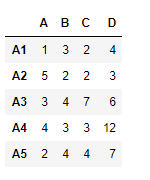Let’s create the series

## Python3

 `# importing pandas as pd``import` `pandas as pd` `# Create the series``sr ``=` `pd.Series([``12``, ``25``, ``64``, ``18``], index ``=``[``"A"``, ``"B"``, ``"C"``, ``"D"``])` `# Print the series``sr`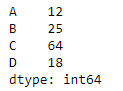Lets use the dataframe.rmul() function to find the multiplication of a series with dataframe

## Python3

 `df.rmul(sr, axis ``=` `1``)`

Output :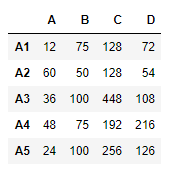Example #2: Use rmul() function to perform multiplication of a dataframe with other.

## Python3

 `# importing pandas as pd``import` `pandas as pd` `# Creating the first dataframe``df1 ``=` `pd.DataFrame({``"A"``:[``1``, ``5``, ``3``, ``4``, ``2``],``                    ``"B"``:[``3``, ``2``, ``4``, ``3``, ``4``],``                    ``"C"``:[``2``, ``2``, ``7``, ``3``, ``4``],``                    ``"D"``:[``4``, ``3``, ``6``, ``12``, ``7``]},``                    ``index ``=``[``"A1"``, ``"A2"``, ``"A3"``, ``"A4"``, ``"A5"``])` `# Creating the second dataframe``df2 ``=` `pd.DataFrame({``"A"``:[``10``, ``11``, ``7``, ``8``, ``5``],``                    ``"B"``:[``21``, ``5``, ``32``, ``4``, ``6``],``                    ``"C"``:[``11``, ``21``, ``23``, ``7``, ``9``],``                    ``"D"``:[``1``, ``5``, ``3``, ``8``, ``6``]},``                     ``index ``=``[``"A1"``, ``"A2"``, ``"A3"``, ``"A4"``, ``"A5"``])` `# Print the first dataframe``print``(df1)` `# Print the second dataframe``print``(df2)`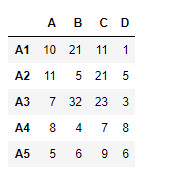Lets perform df2 * df1

## Python3

 `# perform multiplication of df2 with df1``df1.rmul(df2)`

Output :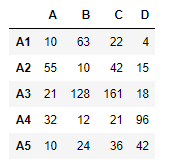My Personal Notes arrow_drop_up